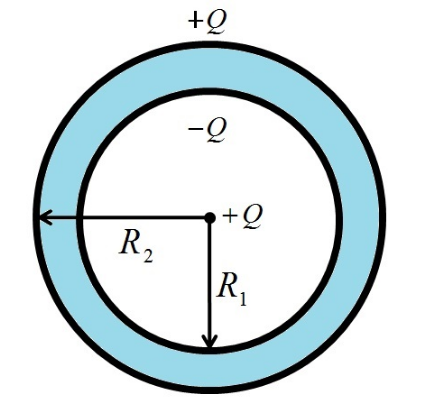Deepak Scored 45->99%ile with Bounce Back Crack Course. You can do it too!

# A metallic spherical shell has an inner radius R1

Question:

A metallic spherical shell has an inner radius R1 and outer radius R2. A charge Q is placed at the centre of the spherical cavity. What will be surface charge density on (i) the inner surface, and (ii) the outer surface?

Solution:

The situation given in question is shown in figure given belowWhen positive charge is placed at the centre of the spherical cavity then an equal amount of negative charge (‒Q) appears on inner surface of the sphere due to induction. This charge is distributed uniformly on the inner surface. Also an equal amount of positive charge (+Q) also appears on outer surface of the sphere.

Now surface charge density on inner surface = (Total charge on inner surface/Area of the inner surface) = ‒Q/(4πR12)

Surface charge density on outer surface = (Total charge on outer surface/Area of the outer surface) = +Q/(4πR22)